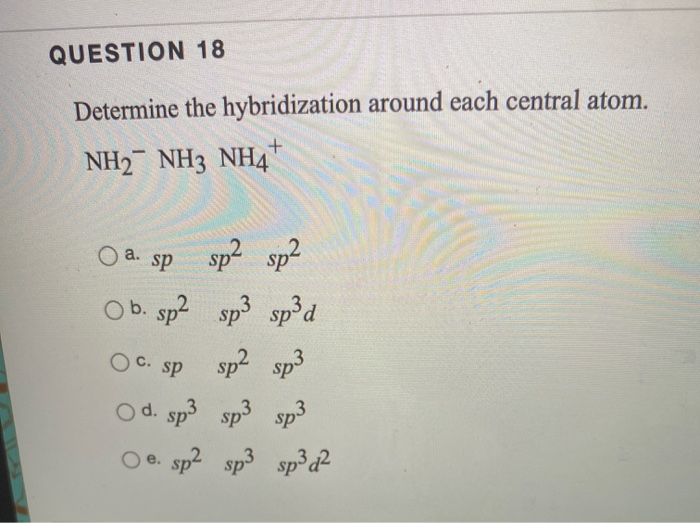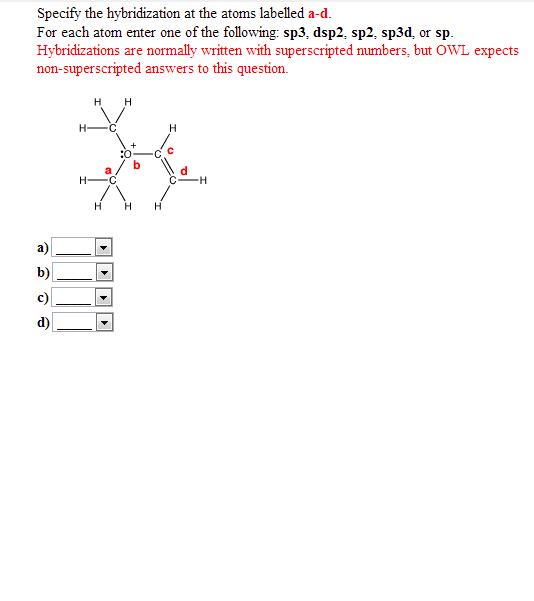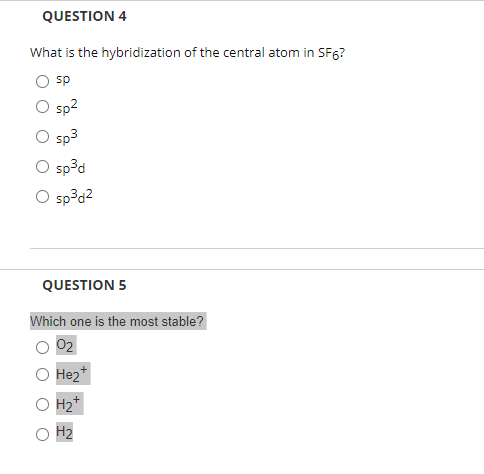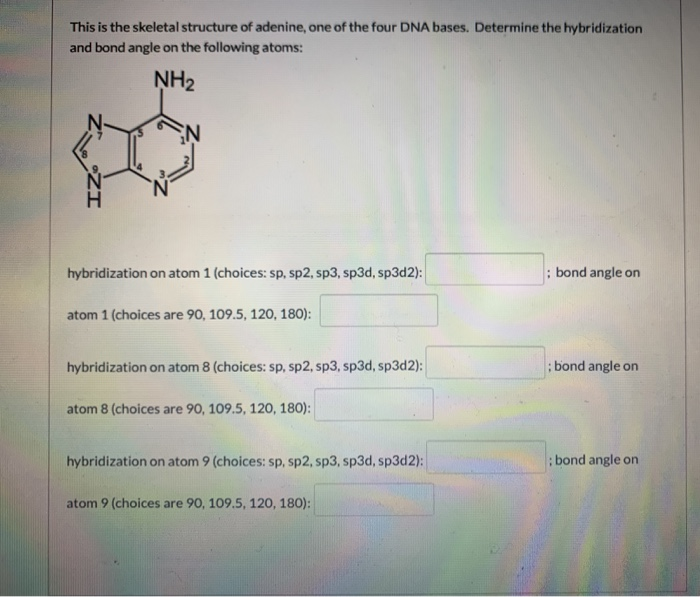Question

What is the hybridization of the central atom of each of the following molecules? Match the...

What is the hybridization of the central atom of each of the following molecules? Match the atoms below with their number---sp, sp2, sp3, sp3d, sp3d2

COH2

H2S

PBr5

Concepts and reason

The hybridization of an organic compound can be explained using the electronic geometry of the compound. Electronic geometry gives information about both bond pair and lone pair of electrons. The geometry of a molecule can be described by Valence Shell Electron Pair Repulsion (VSEPR) theory.

Fundamentals

A Lewis structure is used to determine the electronic geometry as well as molecular geometry of a molecule.

A Lewis structure is a skeletal structure with all the elements and non-bonding electrons shown. Two electrons indicate one electron pair.

The table for hybridization with respect to electronic geometry using different combinations of bonds and lone pairs of electrons present on central atom is below:

 Number of atom/group attached to central atom Number of lone pair of electrons on central atom Electron geometry Hybridization of the central atom 2 0 Linear ${\rm{sp}}$ 3 0 Trigonal planar ${\rm{S}}{{\rm{p}}^{\rm{2}}}$ 2 1 Trigonal planar ${\rm{S}}{{\rm{p}}^{\rm{2}}}$ 4 0 Tetrahedral ${\rm{S}}{{\rm{p}}^3}$ 3 1 Tetrahedral ${\rm{S}}{{\rm{p}}^3}$ 2 2 Tetrahedral ${\rm{S}}{{\rm{p}}^3}$ 5 0 Trigonal bipyramidal ${\rm{S}}{{\rm{p}}^{\rm{3}}}{\rm{d}}$ 4 1 Trigonal bipyramidal ${\rm{S}}{{\rm{p}}^{\rm{3}}}{\rm{d}}$ 3 2 Trigonal bipyramidal ${\rm{S}}{{\rm{p}}^{\rm{3}}}{\rm{d}}$ 2 3 Trigonal bipyramidal ${\rm{S}}{{\rm{p}}^{\rm{3}}}{\rm{d}}$ 6 0 Octahedral ${\rm{S}}{{\rm{p}}^{\rm{3}}}{{\rm{d}}^{\rm{2}}}$ 5 1 Octahedral ${\rm{S}}{{\rm{p}}^{\rm{3}}}{{\rm{d}}^{\rm{2}}}$ 4 2 Octahedral ${\rm{S}}{{\rm{p}}^{\rm{3}}}{{\rm{d}}^{\rm{2}}}$Ans:

$\begin{array}{l}\\{\rm{The}}\,{\rm{hybridization}}\,{\rm{of}}\,{\rm{the}}\,{\rm{central}}\,{\rm{atom}}\,{\rm{of}}\,{\rm{the}}\,{\rm{molecules}}\,{\rm{is:}}\\\\{\rm{CO}}{{\rm{H}}_{\rm{2}}}\,{\rm{ = }}\,{\rm{s}}{{\rm{p}}^{\rm{2}}}\\\\{{\rm{H}}_{\rm{2}}}{\rm{S = s}}{{\rm{p}}^{\rm{3}}}\\\\{\rm{PB}}{{\rm{r}}_{\rm{5}}}{\rm{ = s}}{{\rm{p}}^{\rm{3}}}{\rm{d}}\\\end{array}$

Earn Coins

Coins can be redeemed for fabulous gifts.

Similar Homework Help Questions
• What is the hybridization of the central atom in each of the following? 1.          ...

What is the hybridization of the central atom in each of the following? 1.                             [ Select ]                       ["sp3d", "sp3", "sp3d2", "sp", "sp2"]         beryllium chloride 2.                             [ Select ]                       ["sp3d", "sp3", "sp2", "sp", "sp3d2"]         nitrogen dioxide 3.                             [ Select...

• For the following molecules determine the electron domain hybridization on the central atom and...

For the following molecules determine the electron domain hybridization on the central atom and match with the orbital hybridization. (each hybridized orbital may beused more than once, and some might not be used at all)...... XeCl2..... COF2.....PCl4F.....SF6.....C2Br2.....SiF4.....SF4Answers:A. SP3d3B. SP4C. SP3d2D. SP3dE. SP3F. SP2G. SP

• What is the hybridization of the central atom in a molecule with a square-planar molecular geometry...

What is the hybridization of the central atom in a molecule with a square-planar molecular geometry ? sp sp2 sp3 sp3d sp3d2

• What is the hybridization of the central atom in a molecule with a square-planar molecular geometry...

What is the hybridization of the central atom in a molecule with a square-planar molecular geometry ? Group of answer choices sp sp2 sp3 sp3d sp3d2

• What is the hybridization of the central atom in a molecule with a square-planar molecular geometry...

What is the hybridization of the central atom in a molecule with a square-planar molecular geometry ? Group of answer choices sp sp2 sp3 sp3d sp3d2

• What is the hybridization at the two central carbon atoms of 2-butene? A. sp B. sp2...

What is the hybridization at the two central carbon atoms of 2-butene? A. sp B. sp2 C. sp3 D. sp3d E. sp3d2

• QUESTION 18 Determine the hybridization around each central atom. NH2- NH3 NH4+ O a. sp sp2...QUESTION 18 Determine the hybridization around each central atom. NH2- NH3 NH4+ O a. sp sp2 sp2 OC. Sp O b. sp2 sp3 sp3d sp2 sp3 O d. sp3 o e. sp2 sp3 sp3 d2

• #1. #2. #3. Specify the hybridization at the atoms labelled a-d. For each atom enter one...#1. #2. #3. Specify the hybridization at the atoms labelled a-d. For each atom enter one of the following: sp3: dsp2: sp2: sp3d; or sp. Hybridizations are normally written with superscripted numbers, but OWL expects non-superscripted answers to this question. Specify the hybridization at the atoms labelled a-d. For each atom enter one of the following: sp3: dsp2: sp2: sp3d; or sp. Hybridizations are normally written with superscripted numbers, but OWL expects non-superscripted answers to this question. Specify the hybridization...

• QUESTION 4 What is the hybridization of the central atom in SF6? sp sp2 sp3 spd...QUESTION 4 What is the hybridization of the central atom in SF6? sp sp2 sp3 spd O sp3d2 QUESTION 5 Which one is the most stable? 02 Hez H2+ H2

• 1. 2. This is the skeletal structure of adenine, one of the four DNA bases. Determine...1. 2. This is the skeletal structure of adenine, one of the four DNA bases. Determine the hybridization and bond angle on the following atoms: NH2 Ņ ZI hybridization on atom 1 (choices: sp, sp2, sp3, sp3d, sp3d2); : bond angle on atom 1 (choices are 90, 109.5, 120, 180): hybridization on atom 8 (choices: sp, sp2, sp3, sp3d, sp3d2); bond angle on atom 8 (choices are 90, 109.5, 120, 180): hybridization on atom 9 (choices: sp, sp2, sp3, sp3d,...